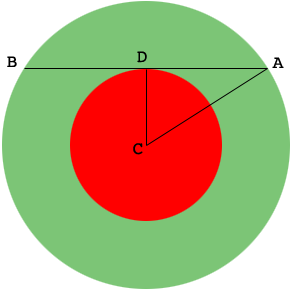SEARCH HOMEMath Central Quandaries & QueriesQuestion from Arman: Two concentric circles have their centre's at point C. The radius of the smaller circle is 8 cm. The length of chord AB is 26 cm and is tangent to the smaller circle. What is the circumference of the larger circle?Hi Arman,

I drew a diagram of what you described and added a point $D$ where the chord is tangent to the smaller circle.It looks like $D$ is the midpoint of $AB.$ Can you prove that it is? What is the length of $AC?$

PennyMath Central is supported by the University of Regina and The Pacific Institute for the Mathematical Sciences.